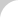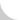The Complete Guide to Chain# 4.1.5 Hanging Transmission Chain Selection

Calculate the chain tension on both the load side and the driving side, and select a chain with a suitable maximum allowable tension to satisfy the requirements. The points of notice are shown below.

• If there are any laws or guidelines for chain selection, check and calculate accordingly. Make sure to follow the manufacturer's selections, and select the safer one of the two selections.
• The chain speed should be less than 50 m/min. If it is more than 50 m/min., consult the manufacturer.
• Use tap fit connecting links that have a tight interference fit.
When you want to use normal connecting links or offset links, you must apply the appropriate derating value (Basics Section 2.2.3) to the chain strength.
• Lubricate the chain joints as much as possible after you reduce the loads.
Lubrication is also required at terminal connections, etc.
• Make sure to follow proper safety procedures, including:
1. Be sure that no one is under the suspended objects.
2. Install a reliable safety guard to avoid damage in the event of chain failure.
3. Examine chains regularly, and replace when necessary.

Figure 4.12 shows some common examples of hanging use. Selection is done according to the flow chart in Figure 4.13.Figure 4.12 Typical Configurations for Hanging ChainFigure 4.13 Chain Selection Procedure (Hanging Chain)Figure 4.14 Example of a Hanging Chain Machine

EXAMPLE: You are planning to use a hanging transmission machine like the one shown in Figure 4.14. Determine if you can use SUPER120 for hanging and SUPER100 for the drive chain. The power source is a 3.7-kW motor (with brake). The motor shaft rotational speed is 1,500 rpm.

• Step 1. Check the motor characteristics.
Rated torque: Tn = 0.038 kN • m
Starting torque: Ts = 0.083 kN • m
Braking torque: Tb = 0.096 kN • m
Motor moment of inertia: Im = 0.015 kN • m
• Step 2. Calculate the chain tension based on load.
The chain tension Fw = M = 3,000 × 9.80665 × 10-3 = 29.4 kN
Service factor Ks = 1.3 (with some shock)
The chain speed coefficient Kv = 1.02 (from the chain speed 6.2 m/min.)
Coefficient for number of sprocket teeth Kc = 1.28 (14-tooth sprocket)
Coefficient of unbalanced load Ku = 0.6 (two sets of chains)
Determine the chain design tension
F′w = Fw × Ks × Kv × Kc × Ku
= 29.4 × 1.3 × 1.02 × 1.28 × 0.6 = 29.9 kN
• Step 3. Calculate the chain tension based on the motor loading. Calculate moment of inertia of motor shaft.

I = M × (V / 2πn1)2 = 3,000 × (6.2 / (2 × π × 1,500))2 = 0.0013 kg • m2

Moment of inertia of motor Im = 0.015 kg • m2
Inertia ratio R = I / Im = 0.087

When there is no play in the system, the coefficient of shock K = 0.23
(Figure 4.7). The chain tension from the starting torque:
Fms = Ts × i × (30 / 14) × 1,000 / (d / 2)
= 0.083 × 60 × (30 / 14) × 1,000 / (171.22 / 2) = 124.7 kN

The chain tension calculated from the breaking torque:
Fmb = Tb × i × (30 / 14) × 1,000 × 1.2 / (d / 2)
= 0.096 × 60 × (30 / 14) × 1,000 × 1.2 / (171.22 / 2) = 173.0 kN

Use the larger value (in this case it is Fmb) to calculate chain tension.
F′mb = Fmb × Kv × Kc × Ku × K
= 173.0 × 1.02 × 1.28 × 0.6 × 0.23 = 31.2 kN

• Step 4. Calculate the chain tension from motor acceleration and deceleration.

Working torque Tm = (Ts + Tb) / 2 = (0.083 + 0.096) / 2 = 0.0895 kN • m

Load torque TL = (M × d) / (2 × 1,000 × i) × (g / 1,000)

= (3,000 × 171.22) / (2 × 1,000 × 60 × (30 / 14)) × (g / 1,000) = 0.02 kN • m

Motor acceleration time ts = ((Im + Il) × n1) / (375 × (Tm − Tl)) × (g / 1,000) × 4

= ((0.015 + 0.00130) × 1,500) / (375 × (0.0895 − 0.02)) × (g / 1,000) × 4 = 0.037s

Motor deceleration time tb = ((Im + Il) × n1) / (375 × (Tm + Tl)) × (g / 1,000) × 4

= ((0.015 + 0.00130) × 1,500) / (375 × (0.0895 + 0.02)) × (g / 1,000) × 4 = 0.023s

Because tb is smaller than ts, the chain tension due to motor deceleration Fb is greater than that of the acceleration.

Fb = (M × V) / (tb × 60 × 1,000) + Fw = (3,000 × 6.2) / (0.023 × 60 × 1,000) + 29.4 = 42.9 kN

Therefore, the chain design tension:
F′b = Fb × Kv × Kc × Ku = 42.9 × 1.02 × 1.28 × 0.6 = 33.6 kN

When comparing the calculated chain tensions in Steps 2, 3, and 4, note that F′b in Step 4 is the greatest. In this tension, Ku is already counted. Comparing F′b with the maximum allowable tension of SUPER 120 chain, F′b < 39.2 kN. Therefore, this chain may be selected.

The example shown above is for chain in hanging drives. The maximum tension on the wrapping transmission chains is:

F′b × d / d′ = 33.6 × 171.22 / 303.75 = 18.9 kN

This value is less than the maximum allowable tension of SUPER 100 chain, which is 30.4 kN. Therefore, this chain is acceptable.

Other Important Considerations

NOTE 1: If there are laws or regulations for chain selection, you must take them into account. For example, if the safety guideline says, "Safety factor must be greater than 10:1 compared with the minimum tensile strength," then you should design the equipment as shown above, and consider the following:

For hanging drive chain:
Minimum tensile strength
= M × g × Ku × 10 = 3,000 × (9.80665 × 10-3) × 0.6 × 10 = 176.5 kN

But the minimum tensile strength of SUPER 120 chain is only 124.6 kN, which is not enough to meet this requirement. Instead, select SUPER 140 chain (213 kN).

Wrapping transmission chain requires more than 99.5 kN of minimum tensile strength, therefore you may select SUPER 100 chain (111 kN).

Regulations are not always safer than manufacturer's suggested selection procedure. Choose the safest system possible.

NOTE 2: If a load greater than the motor braking torque very 48 occasionally occurs, the chains will be subjected to the following loads:

Wrapping transmission chain:

Fd = (0.096 × 1,000 × 60 × 2) / 142.68 × 0.6 = 48.4 kN

Hanging drive chain:

48.4 × (303.75 / 171.22) = 85.9 kN

To avoid chain plastic deformation, the minimum tensile strength must be more than twice these loads (see Basics Section 2.1.1), therefore, you should select SUPER 100 chain and SUPER 160 chain.Figure 4.15 Chain Selection Procedure (Conveyor)